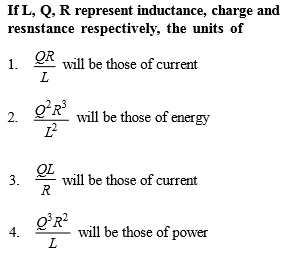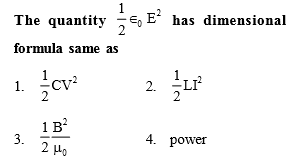[Only for Dropper batch]

Concept Questions :-

Dimensions
High Yielding Test Series + Question Bank - NEET 2020

Difficulty Level:

What are the dimensions of latent heat?

(1) $M{L}^{2}{T}^{-2}$

(2) $M{L}^{-2}{T}^{-2}$

(3) ${M}^{0}L{T}^{-2}$

(4) ${M}^{0}{L}^{2}{T}^{-2}$

Concept Questions :-

Dimensions
High Yielding Test Series + Question Bank - NEET 2020

Difficulty Level:[Only for dropper batch]

Concept Questions :-

Dimensions
High Yielding Test Series + Question Bank - NEET 2020

Difficulty Level:

The dimensional formula of pressure is

1. $\left[{\mathrm{MLT}}^{-2}\right]$

2. $\left[{\mathrm{ML}}^{-1}{\mathrm{T}}^{2}\right]$

3. $\left[{\mathrm{ML}}^{-1}{\mathrm{T}}^{-2}\right]$

4. $\left[{\mathrm{MLT}}^{2}\right]$

Concept Questions :-

Dimensions
High Yielding Test Series + Question Bank - NEET 2020

Difficulty Level:

Light year is a unit of

(1) Time

(2) Mass

(3) Distance

(4) Energy

Concept Questions :-

Dimensions
High Yielding Test Series + Question Bank - NEET 2020

Difficulty Level:

The magnitude of any physical quantity :

(1) Depends on the method of measurement

(2) Does not depend on the method of measurement

(3) Is more in SI system than in CGS system

(4) Directly proportional to the fundamental units of mass, length and time

Concept Questions :-

Dimensions
High Yielding Test Series + Question Bank - NEET 2020

Difficulty Level:

Which of the following is not equal to watt ?

(1) Joule/second

(2) Ampere $×$ volt

(3) (Ampere)2 $×$ ohm

(4) Ampere/volt

Concept Questions :-

Dimensions
High Yielding Test Series + Question Bank - NEET 2020

Difficulty Level:

Newton–second is the unit of

(1) Velocity

(2) Angular momentum

(3) Momentum

(4) Energy

Concept Questions :-

Dimensions
High Yielding Test Series + Question Bank - NEET 2020

Difficulty Level:

Which of the following is not represented in correct unit

(1) $\frac{\text{Stress}}{\text{Strain}}=N\text{​}/\text{​}{m}^{2}$

(2) Surface tension = $N\text{​}/\text{​}m$

(3) Energy $=kg\text{​-​}m\text{​}/\text{​}\mathrm{sec}$

(4) Pressure $=N\text{​}/\text{​}{m}^{2}$

Concept Questions :-

Dimensions
High Yielding Test Series + Question Bank - NEET 2020

Difficulty Level:

One second is equal to :

(1) 1650763.73 time periods of $Kr$ clock

(2) 652189.63 time periods of $Kr$ clock

(3) 1650763.73 time periods of $Cs$ clock

(4) 9192631770 time periods of $Cs$ clock

Concept Questions :-

Dimensions